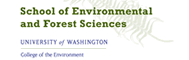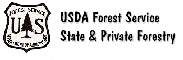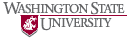The Rural Technology Initiative ceased operations in 2011. This site is maintained as an archive of works from RTI collaborators from 2000 to 2011 and is no longer updated.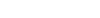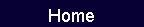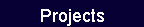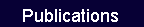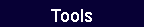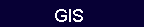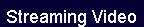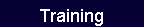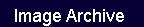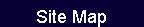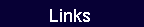# Chapter 7.  Chips, Sawdust, Planer Shavings, Bark, and Hog Fuel

### Residue Calculation Examples

#### Estimating a Hog Fuel Mix

 Chapter 7.  Chips, Sawdust, Planer Shavings, Bark, and Hog Fuel

Previous chapters have presented material balances that can be used to estimate the fraction of a log recovered in a residue form. There are many factors that affect chip and residue measures and associated conversion factors. These include:

•   Size and geometry: The differences in physical characteristics of these residues are obvious and affect how they occupy a unit of space.

•   Specific gravity: Wood and bark densities differ between species (Tables 1-1, 7-5).

•   Moisture content: Wood and bark moisture contents vary between species (Table 1-1), and water may be added during pond storage and debarking. Buyers and sellers of residues and statistical reporting organizations generally devise some method for correcting to the oven-dry state.

•   Degree of compaction: Over time, chips and residues will settle due to gravity. Also, mechanical and pneumatic compaction can be used to pack more residue into a given space.

Quantities of chips and residues are measured in units of volume or weight. This chapter discusses volumetric expansion factors and bulk densities for these products, typical units of measure and conversion, and residue yields, and also presents examples that integrate this material.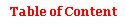### Expansion Factors, Relative Solid Volume, and Compaction

To illustrate these terms, consider a log con­taining 5 cubic feetof solid wood (Vsw) that is chipped. Obviously, the chips will occupy more space than 5 cubic feet.

Expansion (Fluffing) Factor

Loose Expansion Factor.     Immediately after chipping, suppose the loose chips occupy 15 cubic feet (Vp). The expansion factor (E), also called the fluffing factor, is

E (loose)  =  Vp  /  Vsw  =  15 ft3  /  5 ft3  =  3.00.

Note that when Vsw and Vp are measured in cubic meters, the expansion factor has the same value.

Compacted Expansion Factor.     Loose chips will settle over time due to gravity, or they may be physically compacted by equipment. Suppose the chips are compacted so the space occupied (Vp) reduces to 12 cubic feet. The expansion factor is

E (compacted)  =  12 ft3  /  5 ft3  =  2.40.

These calculations illustrate the importance of noting the degree of compaction associated with a particular expansion factor. Measures of compaction are discussed below.

Relative Solid Volume

The reciprocal of the expansion factor measures the number of solid cubic feet (cubic meters) that will yield a cubic foot (cubic meter) of residue. Continuing the example, relative solid volume (RSV), also termed volume occupancy, is

RSV   (loose)   =  1  /  E  (loose)
=   1  /  3. 00  =  0.333.

RSV   (compacted)  =   1  /  E  (compacted)
=   1  /  2.40  =  0.417.

Some multiply RSV by 100 to express it as a percentage; in this form it is sometimes called a compaction rate.

Compaction

Compaction Ratio.     A common measure of compaction is the compaction ratio (CR), which is

CR     =  Vp  (loose)  /  Vp (compacted)
=   15 ft3  /  12 ft3  =  1.25.

CR     =   E  (loose)   /  E (compacted)
=   3.00  /  2.40  =  1.25.

CR can also be calculated from the relative solid volumes. It has a value of one for loose residue and increases as the particles become more compacted. A maximum value for CR can be estimated if it is assumed that the maximum compaction possible would compress the residue to the original volume of solid wood. Under these conditions:

Vp  (compacted)  =  Vsw
hence
CR  =   15 ft3  /  5 ft3  =  3.00.

In other words, the limiting value of the compac­tion ratio is numerically equal to the expansion factor for loose particles.Compaction Percent.     A less common compaction value can be obtained from volume changes. From the example, the maximum compaction from loose chips to solid wood is 10 cubic feet, while the actual compaction is 3 cubic feet. Actual compaction as a percentage of the maximum possible is

Compaction %  =  100  *  [Vp (loose) -  Vp                                      (compacted)]  /  [Vp (loose) -  Vsw]
=  100  *  (15 - 12)  /  (15 - 5)  =  30%.

Summary

Table 7-1 presents expansion factors for various types of residues and corresponding relative solid volumes. Also shown are conversions from Imperial to metric and equivalents when residues are meas­ured in 200 cubic feet volumetric units (see p. 87). Tables 7-2 and 7-3 present additional sources of expansion factor and compaction ratios. The con­version methods applied to the expansion factors in Table 7-1 can also be applied to the expansion factors presented in Table 7-2.

Bulk Density

Bulk density (BD) refers to residue weight divided by residue volume. Suppose the example log has a moisture content of 80% MCod (44.4% MCw)and specific gravity (SGg)  is 0.48 (see Chapter 1 for definitions). Using methods pre­sented in Chapter 1, wood density is 53.9 wet pounds per green cubic foot. This is composed of 29.9 lb of oven-dry wood plus 24.0 lb of water. The term basic density is sometimes used to refer to the oven-dry weight per cubic foot (i.e., 29.9 lb/ft3). The chips from the 5 cubic-foot log have the following weight distribution:

 Condition Weight  (lb) Percent Oven-dry wood  (Wod) 149.5 55.6 Water  (MCw) 120.0 44.4 Wood + water  (Wwet) 269.5 100.0

The term solids fraction refers to the percentage of total weight that is oven-dry wood.

As was described for solid wood density in Chapter 1, bulk density can be calculated for any combination of numerator (weight) and denomi­nator (volume) moisture contents, hence it is important to specify these conditions. The more common cases are given in the remainder of this section.Oven-dry Bulk Density

This is the oven-dry weight per green cubic foot of residue. Divide the weight of oven-dry wood (Wod) by the residue volume (Vp)

BDod (loose)  =  Wod  /  Vp  (loose)
=  149.5 lb  / 15 ft3  =  10.0.

BDod (compacted)  =  Wod  /  Vp (compacted)
=  149.5 lb  /  12 ft3  =  12.5.

The same results can also be obtained by dividing the basic density (29.9 lb/ft3) by the appropriate expansion factor.

These bulk densities indicate the amount of oven-dry wood present in each loose or compacted green cubic foot of residue. They are important in residue transactions because purchasers wish to pay only for wood and therefore want the weight of water excluded. The residue volume is green, since these are undried chips fresh from the log. In cases where residues are dried or manufactured from dry wood, these bulk densities would change because wood shrinkage will modify the volume the residue occupies. This will be illustrated below.

Wet Bulk Density

Bulk density can also be calculated with the moisture content included in the weight (total weight per green cubic foot of residue):

BDwet (loose)  =  Wwet  / Vp  (loose)
=  (269.5 lb  /  15 ft3)  =  18.0.

BDwet (compacted)  =  Wwet  /  Vp
(compacted)   =  (269.5 lb  /  12 ft3)  =  22.5.

The same results can also be obtained by dividing the wet wood density (53.9 lb/ft3) by the appropriate expansion factor. These values are useful for estimating actual weights and loadings on equipment.

For the same compaction, knowledge of moisture content allows conversion between BDod and BDwet:

BDwet  =  BDod  *  (1 + MCod / 100).

Effect of Drying (Shrinkage)on Bulk Density

Bulk densities of dry residues or residues manufactured from dry wood will differ from those in the preceding example. One reason is that the type of residue may be different. Examples are planer shavings and sander dust obtained when kiln-dried wood is surfaced; these residue forms may have different expansion factors. Another reason is that because wood shrinks as it dries, the residue occupies less space. Since many secondary wood products industries, such as millwork and furniture, utilize dry wood, their oven-dry bulk densities will be higher and their wet bulk densi­ties will be lower due to the effects of shrinkage and lower moisture content. See Example 1.

Summary

This section has demonstrated how various factors affect bulk density calculations. Tables 7-2 and 7-3 present data illustrating the influence of mill source (residue type) and species on bulk densities. It should be obvious from the discussion that bulk density is ambiguous without clear statement of the species (wood specific gravity), type of residue, degree of compaction, and the moisture content of both the numerator and denominator.Units of Residue Measure and Conversions

The 200 Cubic Foot Volumetric Unit

A unit representing 200 cubic feet of space is a very common measure for residues. It is sometimes referred to as a gravity packed unit (GPU). Care should be taken when the word unit is used, since it may refer to other measures (e.g., bone-dry unit in the next section).

Example  1

Suppose 5 cubic feet of solid green wood is dried to 10% MCod (9.1% MCw) and that the species has SGg  =  0.48 and 15% total volumetric shrinkage.

As seen in Chapter 1, shrinkage reduces the volume of solid wood to 4.5 cubic feet. If this dry wood is chipped with the same equipment that converted the green log into 15 cubic feetof green chips, the expansion factor of 3.00 predicts that the loose dry chips will occupy 13.5 cubic feet. The same result is obtained by applying volumetric shrinkage to the drying of the green chips to 10% MCod. If the dry chips are compacted to the same degree as the green chips, the compacted volume is 10.8 cubic feet.

Using the methods explained in Chapter 1, we will find the weight density of this dry wood to be 36.55 lb/ft3. Of this, 33.2 pounds are oven-dry wood and 3.35 pounds are water. The weight distribution of the dry chips is

 Condition Weight (lb) Percent Oven-dry wood  (Wod) 149.5 90.9 Water  (MCw) 5.0 9.1 Wood + water  (Wwet) 164.5 100.0

Note that the solids fraction has risen to 90.9%. Using the previously calculated expansion factor for loose chips, bulk densities are

BDod     (loose)  =  149.5 lb  /  13.5 ft3  =  11.1.

BDwet   (loose)  =  164.5 lb  /  13.5 ft3  =  12.2.

Calculations for compacted chips are similar except the compacted chip volume of 10.8 cubic feetis substituted. These results can also be obtained by dividing "basic" and wet wood densities by the loose expansion factor.

Note that the cubic foot volume here refers to volume that remains after shrinkage to 10% MCod rather than the original green volume. The difference between the oven-dry and wet bulk densities has narrowed. The oven-dry bulk density increased because the volume occupied by the dry wood is less. The wet bulk density is also based on the smaller volume occupancy, but this is offset by the great reduction in weight of water. Hence the net effect is for the wet bulk density to decrease.

The solid equivalent of a unit varies depending on particle geometry and degree of compaction. Table 7-1, column 7, divides the expansion factors into 200 to estimate the number of solid cubic feet of wood (or bark) that will expand to fill a unit.

Table 7-3.  Average moisture content (MCw) and bulk density of various wood residues.

 Bulk density (lb/ft3) Green Oven-dry Residue MCw Mean                    SE Mean                    SE U.S. South Hardwood sawdust and bark 45 25.8                      0.30              14.2                    0.11 Mixed pine-hardwood sawdust 41 23.1                      0.44              13.7                    0.31 Clean hardwood pulp chips 43 22.9                      0.55              13.1                    0.58 Hardwood whole-tree chips 35 20.4                      0.11              13.2                    0.06 Pine whole-tree chips 40 18.8                      0.10              11.3                    0.08 Hogged dry trims (hardwood) 12 15.7                      0.40              13.8                    0.23 Shavings (hardwood) 8 10.8                      0.59                9.8                    0.55 Western Oven-dry density (lb/ft3) Hogged fuel 7.9  -  11.4 Sawdust 8.0  -  11.2 Bark 8.5  -  12.6 Secondary mill sawdust 8.8  -  14.0 Shavings 3.8  -   7.9

Sources:  U.S. South: Harris and Phillips (1989).  Western:  Risbrut and Ellis (1981).

SE = standard error.Column 9 presents reciprocals of column 7 (i.e., number of units that will result when one solid cubic foot is converted into the corresponding residue type). Column 8 presents the metric counterpart to column 7 (i.e., the number of solid cubic meters that will expand to fill a cubic meter of space with the particular residue).

Conversions:

1 unit  =  200 ft =  5.66 m3.

1 m3  =  35.315 ft3  =  0.177 unit.

Weight Measures

The common weight measures and conversions used in transactions and in various statistical sources are shown in Table 7-4. The weight of a bone-dry unit (BDU) was obtained by experimen­tally weighing a number of cords of Douglas-fir slab wood that were dried to 3 to 7% moisture content. The average weight, nearly 2,400 pounds, has become a standard basis for measuring residues.

Of these weight measures, the long ton is the least common but is found in some statistical reports. Due to moisture content variation, weights are generally corrected to a zero percent moisture content (oven-dry or bone-dry). Consequently, actual shipping weight will be much greater. Two useful conversions are (Appendix 1, Table 2):

To convert lb/ft3 to kg/m3 multiply by 16.0185.

To convert kg/m to lb/ft3 multiply by 0.062428.

 Table 7-4.  Residue weight measures and conversions. Short Long Oven-dry (lb) Oven-dry (kg) ton ton BDU Tonne Short ton 2,000 907.18 1.000 0.909 0.833 0.907 Long ton 2,200 998.18 1.100 1.000 0.917 0.998 BDU 2,400 1,088.62 1.200 1.091 1.000 1.089 Tonne 2,204.62 1,000.0 1.102 1.002 0.919 1.000

Source: Calculated by the author.

Stowage Factor and Compaction in Shipping

Since wood chips and residues are light in weight, compaction is very important to minimize the transporation cost per ton.

Stowage Factor.     This is a marine term that measures the cubic volume of cargo space for products of known weights. It represents the number of cubic feet required, including void space, to contain a long ton of a product. Therefore, a stowage factor of 120 means that 120 cubic feet of space is needed to stow a long ton of a particular product.

Compaction Percent.     Because of variability in moisture content, stowage factors for chips are not reliable and an alternative, compaction percent, is more commonly used. Compaction means the packing of chips so the voids are minimized. The pulp and paper industry defines compaction as the number of bone-dry units (BDU) that can be placed in a 200 cubic foot space. Thus one faces the po­tentially confusing situation relating BDU (a weight measure) to GPU (a volume measure).

Compaction is usually expressed as a per­centage. A 100% compaction means that a BDU occupies exactly 200 cubic feet, which implies a bulk density of 2,400 lb/200 ft3 = 12 lb/ft3. Similarly, 90% compaction means that 0.90 BDU occupies 200 cubic feet (bulk density  =  10.8 lb/ft3). With modern shiploading equipment, compaction percentages of 100% or more are commonly achieved.

Compaction percent can be calculated by one of the following formulas.

Compaction percent

=  100 * BDU * 200 / ship volume, ft3

=  (long tons loaded) * 1.12 (100 - MCw)

* 200 / (1.2 * ship volume, ft3)

=  2,240 * 200 * (100 - MCw) /

(stowage factor * 2,400)

=  186.6 (100 - MCw) / stowage factor.Residue Yield

Sawdust

The volume of sawdust produced in manu­facturing lumber depends on sawkerf thickness, product line (number of saw cut lines), and log diameter. For a given log size and sawkerf, a mill producing smaller lumber items will generate more sawdust than a mill cutting large timbers. The material balance references in Chapter 4 are a good source of average sawdust yields in solid wood equivalent. The sawdust expansion factor in Table 7-1 or 7-2 should be applied to convert these solid wood equivalents to volume of sawdust as shipped.

Pulp Chips

Pulp chips are obtained from three principal sources: (1) whole logs, (2) sawmill slabs, edgings, and trimmings, and (3) veneer mill scraps and peeler cores, if the latter are not sold to stud sawmills or preservative plants for posts. The material balances in Chapters 4 and 5 provide esti­mates of average chip yields in solid wood equiva­lent from these processes. The chip expan­sion factor in Table 7-1 or 7-2 should be applied to convert these solid wood equivalents to volume of chips as shipped. The material balances assume that residues recovered as chips are fully utilized by pulp mills. Actually, a reject loss on the order of 2 to 5% will occur during pulp chip screening. This reject loss will be at the upper or lower end of this range depending on the condition of the chipper, the chip specification, and whether oversize chips are rechipped.

Planer Shavings

The volume of planer shavings obtained depends on the fraction of lumber output that is planed and the difference between the rough and surfaced dimensions. The material balances and computational methods in Chapter 4 can be used to estimate average shaving yields. Since these material balance estimates are in solid wood form, the expansion factor in Table 7-1 or 7-2 must be applied to get volume as shipped.

Bark

Bark volume varies with species, position on the tree, and tree size. Average bark volume can be estimated either as a percent of total volume (including bark) or as a percent of wood volume:

Bark volume as a percent of total volume
(BTV%)   =   (dob2 - dib2) / dob2  * 100

Bark volume as a percent of wood volume
(BWV%)  =  (dob2 - dib2 ) / dib2 *  100

where   dob  =  diameter outside bark

dib =  diameter inside bark.

For example, for a log measuring 20 inches in diameter inside bark, with bark one inch thick, the bark percentages are

BTV%   =   (222  - 202) / 222  * 100  =  17.4%.

BWV%   =   (222  - 202) / 202  * 100  =  21.0%.

This calculation is likely to overestimate the true bark volume because of fissures and voids in the bark. A range of 26 to 28% void volume was found for three softwood species (Krier and River 1968), hence the above results should be reduced by this amount. The recovered bark may be lower, due to miscellaneous losses during harvesting, log handling, and debarking.

Smith and Kozak (1967) developed bark thickness regressions for a variety of western Canadian species. Table 7-5 presents average total bark volume percentages, moisture contents, and specific gravities of inner and outer bark. The bark moisture contents reflect conditions that would normally be found in logs and do not include additional water from pond storage or water added during debarking.Table 7-5. Bark contents of western species.

 Inner bark Outer bark Bark volume, % of % of percent of total total Species Wood             Total bark            MCod%              SGg bark            MCod%          SGg SOFTWOODS Cedar Western redcedar 16                  14 36               88                   0.36 64               37               0.38 Yellow (Alaska) 15                  13 52             145                   0.41 48               79               0.38 Douglas-fir 28                  22 38             133                   0.45 62               80               0.43 Fir, true 13                  12 65               77                    0.52 35               40               0.58 Hemlock, western 20                  16 54             134                   0.45 46               65               0.56 Pine Lodgepole 11                  10 30             128                   0.34 70               42               0.51 Ponderosa 31                  24 12               78                    0.36 88               21               0.34 Western white 13                  12 23             118                   0.31 77               75               0.54 Spruce Engelmann 15                  13 59             121                   0.45 41               60               0.53 Sitka 9                  9 45             112                   0.44 55               55               0.62 Hardwoods Red alder 16                  14 56               88                    0.52 44               66               0.62 Aspen 20                  17 35             121                   0.37 65               93               0.54 Birch 22                  18 65               68                    0.63 35               22               0.66 Black cottonwood 12                  11 48             130                   0.41 52               77               0.44 Bigleaf maple 19                  16 68             134                   0.66 32               70               0.45

Source: Smith and Kozak (1971).

Hog Fuel

Hog fuel is generally a mix of bark, sawdust, and planer shavings. The relative amount of each depends on a particular mill's marketing alternatives for these residues. The mix can be estimated as a weighted average using the information presented in the appropriate yield sections (see Estimating a Hog Fuel Mix below). If an estimate is needed with a high degree of confidence, samples of the hog fuel in question should be analyzed.Residue Calculation Examples

Estimating Yield from Plywood

The following procedure can be used for lumber, veneer, plywood, and so forth. Assume that it is desired to estimate the number of 200 cubic foot units of chips recovered per 1,000 square feet (3/8 inch basis) of Douglas-fir plywood. The average mill-length block is 15 inches in diameter.

1.   From the plywood material balance studies (Fahey and Willits 1991), about 25% of a mill-length veneer block is recovered as chippable residue, and about 50% is recovered as dry veneer. About 12% of the dry veneer will be lost in making plywood, so about 45% of the block will be plywood.

2.   Chapter 5 shows that 1,000 square feet, 3/8 inch basis, plywood has a cubic volume of 31.25 cubic feet.

3.   Since the cubic volume of plywood (31.25 ft3) represents 45% of the cubic block volume and since the cubic volume of clean chips represents 25% of the block volume, the solid wood equi­valent volume of chips can be estimated by proportions as follows:

45 / 31.25  =  25 / x.

Solving, yields x  =  17.4 ft3 solid equivalent of chips recovered per 1,000 ft2, 3/8 inch, plywood.

4.   Convert the solid chip volume by the compacted chip expansion factor of Table 7-1. Compacted chips

=  17.4 ft3 solid chips * 2.50 ft3compact chips per solid ft3  =  43.5 ft3.

5.   Since a unit is 200 cubic feet, there are 43.5 / 200  =  0.218 units of compact chips recovered per 1,000 square feet of 3/8 inch basis plywood.

Assume a plywood process with the same conditions as indicated above.

1.   From the material balance equations (Fahey and Willits 1991), about 25% of a mill-length veneer block is recovered as chippable residue.

2.   Yield of chips  =  100 ft3/cunit * 0.25  =  25 ft3 solid chips.

3.   Multiply by the compacted chip expansion factor in Table 7-1. Compacted chips  =  25 ft3solid chips * 2.50 ft3 compact chips per ft3 solid chips  =  62.5 ft3.

4.   Therefore, a cunit of veneer blocks yields 62.5 / 200  =  0.3125 units of compact chips.

Converting Units of Residue to Weight

How many units of pulp chips equal an oven-dry short ton?

1.   Assume the species is Douglas-fir, which has a SGg of 0.45 (Table 1-1). Table 1-2 shows the weight density at 0% MCod, or 28.1 pounds per solid cubic foot.

2.   Divide by the compact chip expansion factor (Table 7-1) to get a bulk density of 11.24 oven-dry lb/ft3 compact chips. Alternatively, use a bulk density based on samples.

3.   Divide 2,000 pounds in a short ton by the bulk density estimate to get the number of cubic feet of compacted chips per short ton: 2,000 / 11.24  =  178 ft3.

4.   Since a unit is 200 cubic feet, a short ton represents 178 / 200  =  0.89 units under these conditions.

Note:  Simple division shows that for a 200 cubic foot unit to exactly equal a short ton, the bulk density would be 2,000 / 200  =  10 oven-dry lb/ft3 residue. Similar calculations for other weight measures imply oven-dry bulk densities as follows:

Long ton            11.00

BDU                   12.00

Tonne                11.02.Estimating a Hog Fuel Mix

Assume that the hog fuel for a cunit of Douglas-fir logs processed in a sawmill will contain all of the sawdust, planer shavings, and bark produced by the mill.

1.   From the material balances in Chapter 4 and the bark section in this chapter, assume the follow­ing recoveries as percentages of green wood volume: sawdust, 8%; shavings, 5%; bark, 28%. Reduce the bark fraction by 30% to account for fissures, voids, and processing losses so that the net bark fraction is 20%.

2.   Column 1 of the table below multiplies these recovery percents by the 100 cubic feet in a cunit to give solid volume yields in cubic feet.

3.   Column 2 lists the appropriate residue expan-sion factors from Table 7-1, and column 3 is the expanded residue volume in cubic feet after multiplying column 1 by column 2.

4.   Columns 4 and 5 present MCod and SGg assump­tions. It is assumed that sawdust is generated when processing green wood with an MCod of 80%. The planer shavings are assumed to be generated after kiln drying to an average MCod of 16%. The bark MCod and SGg are weighted averages of inner and outer bark of Douglas-fir from Table 7-5. Columns 6 and 7 are estimates of wet and oven-dry solid wood densities using Table 1-2.

5.   Dividing the solid wood densities of columns 6 and 7 by the expansion factors in column 2 results in residue green and dry bulk densities in columns 8 and 9.

6.   Multiplying the residue volume in column 3 by the green and dry bulk densities in columns 8 and 9 results in green and dry residue weights in columns 10 and 11.

7.   Totals of columns 3, 10, and 11 give the final hog fuel values of interest.

For the conditions assumed here, a cunit of logs yields 92 cubic feet (0.46 unit) of hog fuel having a green weight of 1,767 pounds (green bulk density of 19.2 lb/ft3) and oven-dry weight of 972 pounds (dry bulk density of 10.6 lb/ft3). The hog fuel is equal to 0.40 BDU.

 Residue (1) (2) (3) (4) (5) (6) (7) (8) (9) (10) (11) Sawdust 10 2.50 25 80 0.45 50.6 28.1 20.2 11.2 506 281 Shaving 5 4.00 20 16 0.45 32.6 28.1 8.2 7.0 163 141 Bark 20 2.35 47 100 0.44 54.9 27.5 23.4 11.7 1,098 550 Total 92 1,767 972